Courses
Courses for Kids
Free study material
Offline Centres
More

# Equilibrium Class 11 Notes CBSE Chemistry Chapter 7 (Free PDF Download)Last updated date: 26th Nov 2023
Total views: 675k
Views today: 6.73k

## Revision Notes for CBSE Class 11 Chemistry Chapter 7 (Equilibrium) - Free PDF Download

Chemical equilibrium relates to such a state when the reactants, as well as products, will no longer undergo any further change. In such a case, the rate of forwarding and backward reaction remains the same. The concept of equilibrium becomes important for students to grasp the advanced topics. Class 11 Chemistry chapter equilibrium notes provide a clear discussion on it.

Also, check CBSE Class 11 Chemistry revision notes for other chapters:

Equilibrium Chapter Related Important Study Materials
It is a curated compilation of relevant online resources that complement and expand upon the content covered in a specific chapter. Explore these links to access additional readings, explanatory videos, practice exercises, and other valuable materials that enhance your understanding of the chapter's subject matter.

 Equilibrium Related Other Study Materials Equilibrium Important Questions Equilibrium NCERT Solutions Equilibrium NCERT Exemplar

## Equilibrium Class 11 Notes Chemistry - Basic Subjective Questions

### Introduction:

Chemical equilibrium is an important aspect of chemical and biological processes. When a liquid evaporates in a closed container, molecules with relatively higher kinetic energy escape the liquid surface into the vapour phase and a number of liquid molecules from the vapour phase strike the liquid surface and are retained in the liquid phase. It gives rise to a constant vapour pressure because of an equilibrium in which the number of molecules leaving the liquid equals the number returning to liquid from the vapour. Then the system has reached an equilibrium state at this stage. Thus, at equilibrium, the rate of evaporation is equal to the rate of condensation. It may be represented by

${{H}_{2}}O(l)\rightleftharpoons {{H}_{2}}O(vap)$

The above double arrow indicates that the process is simultaneously going in both directions. The equilibrium mixture means the mixture of reactants and products in the equilibrium state is called an equilibrium mixture.

### Equilibrium in the Physical Process:

The most important and familiar examples are the phase transformation process. Eg.

$solid\rightleftharpoons liquid$

$liquid\rightleftharpoons gas$

$solid\rightleftharpoons gas$

a. Solid-liquid Equilibrium:

At a particular temperature and pressure, the ice and water are in equilibrium. The normal melting point or normal freezing point of the substance is the temperature at which the solid phase and liquid phases are equilibrium for any pure substance at atmospheric pressure. When the system at dynamic equilibrium will follow the conditions:

• Both the opposing processes occur simultaneously

• Both the process occurs at the same rate, so the amount of ice and water remains constant.

b. Liquid-vapour equilibrium:

The number of water molecules from the gaseous state into the liquid state increases until the equilibrium is reached.

i.e rate of evaporation = rate of condensation

${{H}_{2}}O(l)\rightleftharpoons {{H}_{2}}O(vap)$

The equilibrium water pressure means the pressure at which the water molecules remain constant at a given temperature and the vapour pressure of water increases with temperature.  The evaporation of liquid depends on,

• The nature of the liquid

• The amount of liquid

• The applied temperature

For example, in a closed vessel water and water vapour are in equilibrium at atmospheric pressure (1.031 bar) and at ${{100}^{0}}C$. This means that for any pure liquid at one atmospheric pressure (1.0301 bar), then the normal boiling point is said to be the temperature at which liquid and vapours are at equilibrium.

c. Solid-vapour Equilibrium:

The examples of this type of system are considered when solids sublime to vapour phase.

${{I}_{2}}(solid)\rightleftharpoons {{I}_{2}}(vapour)$

$Camphor(solid)\rightleftharpoons Camphor(vapour)$

$N{{H}_{4}}Cl(solid)\rightleftharpoons N{{H}_{4}}Cl(vapour)$

### General Characteristics of Equilibrium Involving Physical Processes:

Following characteristics are common to the system at equilibrium for which the physical processes are discussed above:

• At a given temperature, the equilibrium is possible only in closed systems

• If both the opposing processes occur at the same rate then the system is dynamic at stable conditions.

• In a system, all measurable properties remain constant.

• Equilibrium is characterized by the constant value of one of its parameters given, and then it is attained for a physical process.

### Equilibrium in Chemical Processes:

Compared to physical systems chemical reactions also attain a state of equilibrium. Those chemical reactions can occur both in forward and backward directions. The chemical equilibrium is dynamic in nature when the rate of forward reaction is equal to the rate of backward reaction.

### Reversible Reactions

Consider a general case of a reversible reaction

$A+B\rightleftharpoons C+D$

With passing time, there is a decrease in the concentration of reactants A and B, the increase in the concentration of products C and D which leads to a decrease in the rate of forward reaction and an increase in the rate of backward reaction.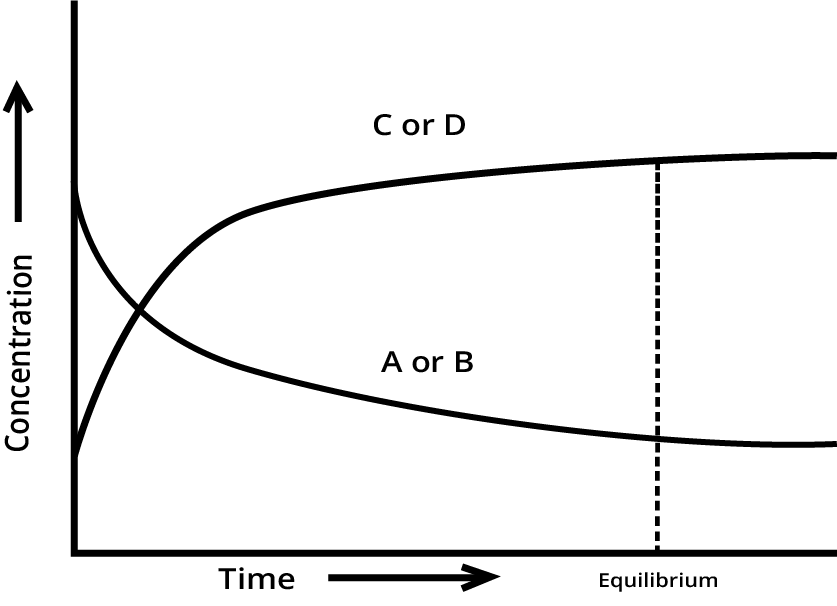When the two reactions occur at the same rate the system will reach a state of equilibrium.

### Irreversible Reactions

The products formed will not react back to form reactants under the same conditions; it is called the Irreversible reaction. These reactions cannot take place in the reverse direction.

$A+B\to C+D$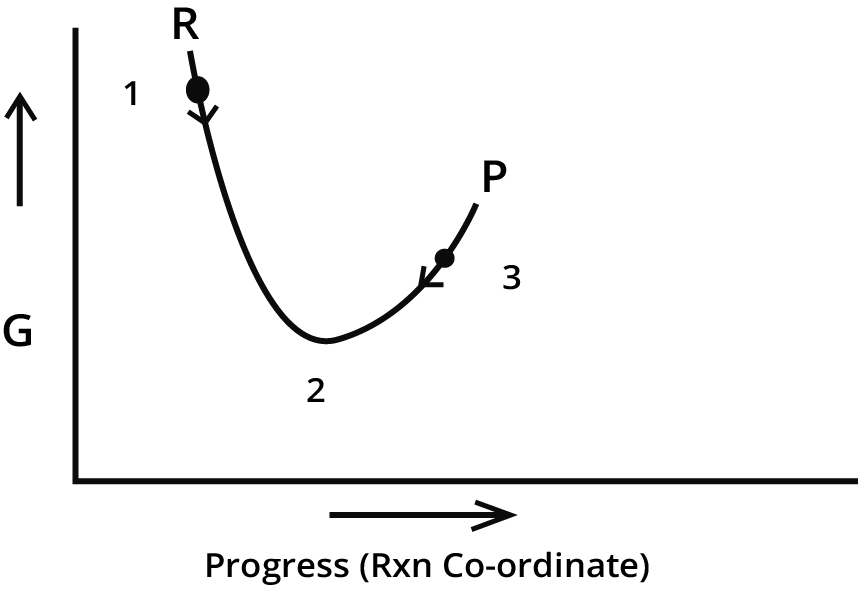• It is a state of minimum Gibb’s energy

• $dG=0 and \Delta G=0$ at this state

• Rate of forward reaction = rate of backward reaction

• This equilibrium is dynamic and stable in nature

### Dynamic Nature of Chemical Equilibrium:

This dynamic nature of chemical equilibrium can be explained by the synthesis of ammonia by Haber’s process. This process starts with definite amounts of ${{N}_{2}}\ and {{H}_{2}}$ and carries out a reaction when equilibrium is attained at a particular temperature. At equilibrium the concentrations of ${{N}_{2}},{{H}_{2}}\ and N{{H}_{3}}$ are constant.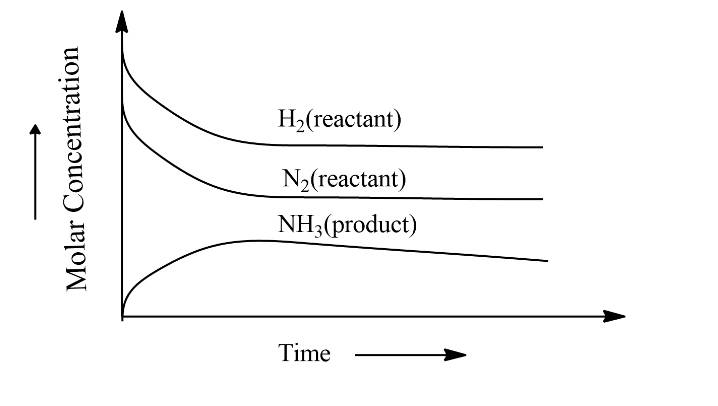### Characteristics of Chemical Equilibrium:

• The concentration of each of the reactants and the products become constant at equilibrium.

• The rate of forward reaction becomes equal to the rate of backward reaction at equilibrium and hence equilibrium is dynamic in nature.

•  None of the products is allowed to escape out or separate out as a solid then only chemical equilibrium can be established.

### Equilibrium Constant:

An equilibrium mixture is a mixture of reactants and products in the equilibrium state.

Consider a general reversible reaction,

$A+B\rightleftharpoons C+D$

Where A and B are reactants, C and D are products in the above-balanced equation.

${{K}_{c}}=\frac{[C][D]}{[A][B]}$

Where ${{K}_{c}}$ is Equilibrium constant and expressed in concentrations of $mol{{L}^{-1}}$.

At a given temperature, the product of concentrations of the reaction products raised to the respective stoichiometric coefficient in the balanced chemical equation divided by the product of concentrations of the reactants raised to their individual stoichiometric coefficients has a constant value. This is known as the Equilibrium Law or Law of Chemical Equilibrium.

The equilibrium constant for the general reaction,

$aA+bB\rightleftharpoons cC+dD$

Equilibrium constant expressed as,

${{K}_{c}}=\dfrac{{{[C]}^{c}}{{[D]}^{d}}}{{{[A]}^{a}}{{[B]}^{b}}}$

Where [A], [B], [C] and [D] are the equilibrium concentrations of the reactants and products.

The product of the molar concentrations of the products, each raised to the power equal to its stoichiometric coefficient is divided by the product of the molar concentrations of the reactants, each raised to the power equal to its stoichiometric coefficient is constant at constant temperature is called Equilibrium Constant.

### Characteristics of Equilibrium Constant:

• The value of the equilibrium constant for a particular reaction is always constant depending only upon the temperature of the reaction and is independent of the concentrations of the reactants with which we start or the direction from which the equilibrium approached.

• The value of the equilibrium constant is inverted when the reaction is reversed.

•  The equilibrium constant for the new equation is the square root of K. (i.e, $\sqrt{K}$) when the equation is divided by 2.

• The equilibrium constant for the new equation is the square of K (i.e, ${{K}^{2}}$) when the equation is multiplied by 2.

• By the addition of a catalyst to the reaction will not affect the value of the equilibrium.

### Predicting the Extent of Reaction:

If the value of ${{K}_{c}}>{{10}^{3}}$ will be high, then the equilibrium reaction is forward dominant.

If the value of ${{K}_{c}}<{{10}^{-3}}$, then the equilibrium reaction is backward dominant.

Moderate value of ${{K}_{c}}$ (between ${{10}^{3}}$  and ${{10}^{-3}}$ ) dominates equilibrium neither directions.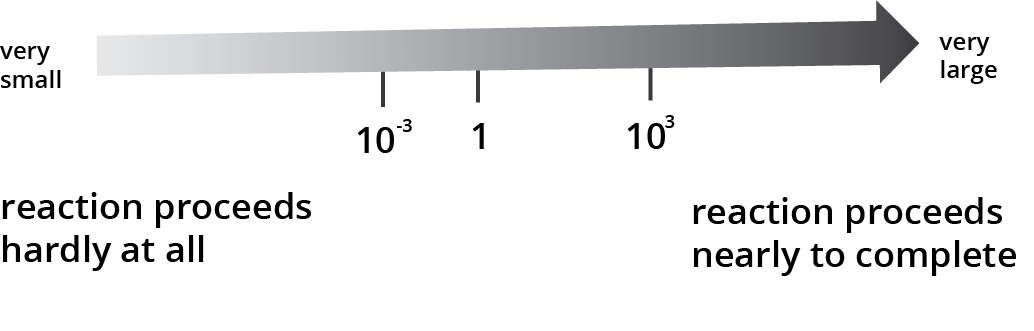### Predicting the Direction of the Reaction- Reaction Quotient:

We can predict the direction in which the reaction will proceed at any stage with the help of the equilibrium constant. Reaction Quotient Q will calculate for this purpose. In the same way as the equilibrium constant ${{K}_{c}}$, Reaction Quotient will not necessarily equilibrium values.

$aA+bB\rightleftharpoons cC+dD$

${{Q}_{c}}=\dfrac{{{[C]}^{c}}{{[D]}^{d}}}{{{[A]}^{a}}{{[B]}^{b}}}$

• the reaction proceeds in the direction of reactants when ${{Q}_{c}}>{{K}_{c}}$

• the reaction proceeds in the direction of products when ${{Q}_{c}}<{{K}_{c}}$

• the reaction mixture is already at equilibrium when ${{Q}_{c}}={{K}_{c}}$

### Calculating Equilibrium Concentrations:

In case of a problem in which we know the initial concentrations but do not know any of the equilibrium concentrations, the following three steps shall be followed:

Step 1. Write the balanced equation for the reaction.

Step 2. Under the balanced equation, make a table that lists for each substance involved in the reaction:

(a) The initial concentration,

(b) The change in concentration on going to equilibrium, and

(c) The equilibrium concentration.

In constructing the table, define x as the concentration (mol/L) of one of the substances that react on going to equilibrium, then use the stoichiometry of the reaction to determine the concentrations of the other substances in terms of x.

Step 3. Substitute the equilibrium concentrations into the equilibrium equation for the reaction and solve for x.

Step 4. Calculate the equilibrium concentrations from the calculated value of x.

Step 5. Check your results by substituting them into the equilibrium equation.

### Relationship Between Equilibrium Constant and Gibbs Free Energy:

The value of the equilibrium constant for a reaction does not depend on the rate of reaction.

• If a change in Gibbs free energy $\Delta G$ is negative, then the reaction proceeds in the forward reaction with spontaneity.

• If a change in Gibbs free energy $\Delta G$ is positive, the products of the forward reaction shall be converted to reactants with a non-spontaneous process.

• If the change in Gibbs free energy is zero, then the reaction is at equilibrium and there is no longer any free energy left to drive the reaction.

A mathematical expression of this thermodynamic view of equilibrium can be described by the following equation:

$\Delta G=\Delta {{G}^{o}}+RT\ln Q$

Where Q is Reaction Quotient. And ${{G}^{o}}$ is standard Gibbs free energy.

At equilibrium, when $\Delta {{G}^{o}}=0$ and ${{Q}_{c}}={{K}_{c}}$

$\Delta G=\Delta {{G}^{o}}+RT\ln {{K}_{c}}=0$

$\Delta {{G}^{o}}=-RT\ln {{K}_{c}}$

$\ln {{K}_{c}}=-\frac{\Delta {{G}^{o}}}{RT}$

$K={{e}^{-\frac{\Delta {{G}^{o}}}{RT}}}$

from the above equation, from the values of $\Delta {{G}^{o}}$ , the reaction spontaneity can be interpreted.

### Homogeneous Equilibria:

In an equilibrium system, all the reactants and products are in the same phase is known as a Homogenous system.

For example,

${{N}_{2}}(g)+3{{H}_{2}}(g)\rightleftharpoons 2N{{H}_{3}}(g)$

In the above reaction, all reactants and products are in the gaseous phase.

$C{{H}_{3}}COO{{C}_{2}}{{H}_{5}}(aq)+{{H}_{2}}O(l)\rightleftharpoons C{{H}_{3}}COOH(aq)+{{C}_{2}}{{H}_{5}}COOH(aq)$

In the above reaction, all reactants and products are homogeneous solution phases.

### Heterogeneous Equilibria:

An equilibrium system having more than one phase is called heterogeneous equilibrium. A familiar example of this type of system is the equilibrium between water vapour and liquid water in a closed container.

${{H}_{2}}O(l)\rightleftharpoons {{H}_{2}}O(vap)$

Similarly, there is an equilibrium between a solid and its saturated solution is a heterogeneous equilibrium.

$Ca{{(OH)}_{2}}(s)\rightleftharpoons C{{a}^{+2}}(aq)+2O{{H}^{-}}(aq)$

### Le Chatelier’s Principle:

This principle helps to decide what course of reaction adopts and make a qualitative prediction about the effect of changes in conditions on equilibrium.

It states that “a change in any of the factors that determine the equilibrium conditions of a system will cause the system to change in such a manner so as to reduce or to counteract the effect of the change.”

This principle is applicable to all physical and chemical equilibrium systems.

a. Effect of Concentration Change:

Generally, equilibrium is disturbed by the addition or removal of any reactant or product. Le Chatelier’s principle predicts that:

• The concentration of added reactant or product is relieved by the direction of the net reaction that consumes the added substance.

• The concentration of removed reactant or product is relieved by the direction of net reaction that replenishes the added substance.

“When the concentration of any of the reactants or products in a reaction at equilibrium is changed, the composition of the equilibrium mixture changes so as to minimize the effect of concentration changes”.

b. Effect of Temperature Change

Because the Reaction Quotient Q no longer equals to the equilibrium constant when equilibrium is disturbed by the change in the concentrations, pressure, or volume and further the composition of the equilibrium mixture changes.

The value of the equilibrium constant changed when a change in the temperature was observed.

Generally, the temperature change dependence of the equilibrium constant depends on the sign of the $\Delta H$ for the reaction.

• If $\Delta H$is negative, then the equilibrium constant of the exothermic reaction decreases because of temperature increases.

• If $\Delta H$ is positive, then the equilibrium constant of the endothermic reaction increases because temperature decreases.

c. Effect of Pressure Change

When the total number of moles of gaseous products is different, then a pressure change obtained changing the volume can affect the yield of products in case of a gaseous reaction. Le-Chatlier’s principle is applied to heterogeneous equilibrium the effect of pressure changes on solids and liquids can be ignored because the volume of a solution or liquid is nearly independent of pressure.

d. Effect of Volume Change

The effect of the increase in pressure is equivalent to the effect of a decrease in volume.

So the effect of a decrease in volume will be shifted towards the equilibrium in the direction in which the number of moles decreases.

e. Effect a Catalyst

The chemical substance increases the rate of a chemical reaction by making available a new low energy pathway for the conversion of reactants to products.

A catalyst increases the rate of forward and reverse reactions that pass through the transition state which does not affect the equilibrium.

For example: contact process

$2S{{O}_{2}}(g)+{{O}_{2}}(g)\rightleftharpoons 2S{{O}_{3}}(g);{{K}_{c}}=1.7\times {{10}^{26}}$

The oxidation of sulphur dioxide to sulphur trioxide is partially very slow.

So, Platinum or divanidium pentaoxide (${{V}_{2}}{{O}_{5}}$) is used as a catalyst to increase the rate of reaction.

f. Effect of Inert Gas Addition

If an inert gas such as argon is added and the volume kept constant this does not take any part in the reaction. Thus, the equilibrium remains undisturbed. Because the addition of inert gas does not change the partial pressures or the molar concentrations of substances involved in the reaction at constant volume.

### Ionic Equilibrium in Solution:

An example of equilibrium reaction that involves ions is given below:

$F{{e}^{+3}}(aq)+SC{{N}^{-}}(aq)\rightleftharpoons [Fe{{(SCN]}^{2+}}(aq)$

Micheal Faraday classified the substances into two categories based on their ability to conduct electricity. Those are electrolytes and non-electrolytes. Because of the presence of the ions, the solution of electrolytes conducts electricity.

For example, an aqueous solution of NaCl is dissociated completely into $N{{a}^{+}}$ and $C{{l}^{-}}$ ions, because of almost 100% ionization in the case of sodium chloride. But in the case of acetic acid which is a weak electrolyte, 5% ionization takes place. Hence, equilibrium is established between ions and unionized molecules in weak electrolytes. This type of equilibrium involving ions in an aqueous solution is known as Ionic Equilibrium.

### Classification of Electrolytes:

Based on the strength of electrolytes are classified into two categories:

a. Strong Electrolytes

The electrolytes which are easily broken into ions with complete dissociation are known as strong electrolytes.

Eg: $HCl,NaCl,NaOH,HN{{O}_{3}},HCl{{O}_{4}},{{H}_{2}}S{{O}_{4}}$

b. Weak Electrolytes

The electrolytes which are partially dissociated into ions are known as weak electrolytes.

Eg:$C{{H}_{3}}COOH,N{{H}_{4}}OH,HCN,{{H}_{2}}{{C}_{2}}{{O}_{4}}$ , and all organic acids and bases.

### Acids, Bases and Salts:

There are various theories from the explanation of acids and bases.

### Arrhenius Concept of Acids and Bases:

According to this theory, acids are the substances that give ${{H}^{+}}$ ions and bases which produce hydroxyl ions $O{{H}^{-}}$ in water.

### Arrhenius Acids:

According to Arrhenius, acids increases the ${{H}^{+}}$ ion concentration in water.

The ionization of acids can be represented by the following equations for HX (aq):

$HX(aq)\to {{H}^{+}}(aq)+{{X}^{-}}(aq)$

Or

$HX(aq)+{{H}_{2}}O(l)\to {{H}_{3}}{{O}^{+}}(aq)+{{X}^{-}}(aq)$

Example: $HCl,{{H}_{2}}S{{O}_{4}},C{{H}_{3}}COOH$ etc.

### Arrhenius Bases:

According to Arrhenius, bases increases the $O{{H}^{-}}$ ions concentration in water.

For example: $NaOH,Ca{{(OH)}_{2}},N{{H}_{4}}OH$ etc.

MOH like base molecule ionizes in aqueous solution from the following equation:

$MOH(aq)\to {{M}^{+}}(aq)+O{{H}^{-}}(aq)$

### The Bronsted-lowry Acids and Bases:

According to Brönsted-Lowry theory, acid is a substance that is capable of donating a hydrogen ion H+ and bases are substances capable of accepting a hydrogen ion, H+. In short, acids are proton donors and bases are proton acceptors.

For example, consider the dissolution of $N{{H}_{3}}$ in ${{H}_{2}}O$ represented by the following equation: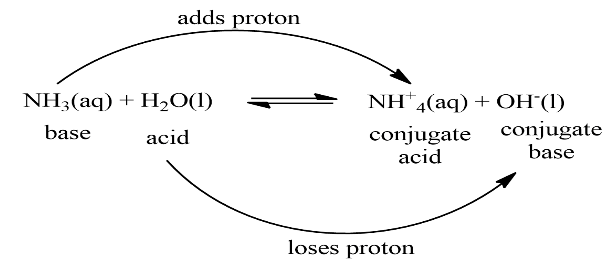In this reaction, a water molecule that acts as a proton donor is known as Lowry-Bronsted acid and an ammonia molecule that acts as a proton acceptor is known as Lowry-Bronsted base.

### Lewis Acids and Bases:

According to this theory, acid is a species that accepts electron pairs and a base that donates electron pairs.

A typical example is a reaction of electron-deficient species $B{{F}_{3}}$ with $N{{H}_{3}}$.

$B{{F}_{3}}+:N{{H}_{3}}\to B{{F}_{3}}:N{{H}_{3}}$

Lewis acids are electron deficient species like $AlC{{l}_{3}},C{{o}^{+3}},M{{g}^{+2}}$

Lewis bases are electron pair donating species like ${{H}_{2}}O,N{{H}_{3}},O{{H}^{-}}$

### The Ionization Constant of Water and its Ionic Product:

Some substances have the unique ability to act as acid and base like water molecules.

${{H}_{2}}O(l)(acid)+{{H}_{2}}O(l)(base)\rightleftharpoons {{H}_{3}}{{O}^{+}}(aq)+O{{H}^{-}}(aq)$

The dissociation constant is represented by:

$K=\frac{[{{H}_{3}}{{O}^{+}}][O{{H}^{-}}]}{[{{H}_{2}}O]}$

The concentration of water is neglected from the denominator as water is pure liquid and its concentration remains constant. Then the equilibrium constant is known as the Ionic Product Of Water, ${{K}_{w}}$.

${{K}_{w}}=[{{H}^{+}}][O{{H}^{-}}]$

The concentration of ${{H}^{+}}$ = $1.0\times {{10}^{-7}}M$ at 298K

As dissociation of water produces equal number of $[{{H}^{+}}]=[O{{H}^{-}}]=1\times {{10}^{-7}}M$

${{K}_{w}}=[{{H}_{3}}{{O}^{+}}][O{{H}^{-}}]={{(1\times{{10}^{-7}})}^{2}}=1\times {{10}^{-14}}{{M}^{2}}$

We can distinguish acidic, neutral, and basic solutions by the relative values of the ${{H}_{3}}{{O}^{+}}$ and $O{{H}^{-}}$ concentrations:

Acidic: $[{{H}_{3}}{{O}^{+}}]>[O{{H}^{-}}]$

Neutral: $[{{H}_{3}}{{O}^{+}}]=[O{{H}^{-}}]$

Basic: $[{{H}_{3}}{{O}^{+}}]<[O{{H}^{-}}]$

### The pH Scale:

Hydronium ion concentration in molarity is more conveniently expressed on a logarithmic scale known as the pH Scale.

From the definition of pH,

$pH=-\log [{{H}^{+}}]$

at ${{25}^{o}}C$ pure water has a concentration of hydrogen ions, $[{{H}^{+}}]={{10}^{-7}}M$

Hence, the pH of pure water is given as:

$pH=-\log ({{10}^{-7}})=7$

Acidic solutions posses a concentration of hydrogen ions, $[O{{H}^{-}}]>{{10}^{-7}}M$ , while basic solution posses a concentration of hydrogen ions, $[O{{H}^{-}}]<{{10}^{-7}}M$

Thus, acidic solution has pH < 7

Basic solution has pH < 7

Neutral solution has pH = 7

Then the ionic product of water, ${{K}_{w}}=[{{H}_{3}}{{O}^{+}}][O{{H}^{-}}]={{10}^{-14}}$

Taking negative logarithms on both sides of equation, we obtain

$-\log {{K}_{w}}=-\log \{[{{H}_{3}}{{O}^{+}}][O{{H}^{-}}]\}=-\log {{10}^{-14}}$

$p{{K}_{w}}=pH+pOH=14$

### Ionization Constants of Weak Acids:

Consider a weak acid HX which is partially ionized in the aqueous solution. The equilibrium can be expressed by:

$\underset{\text{Initial concentration}}{\mathop{{}}}\,\underset{0}{\mathop{HX(aq)}}\,+{{H}_{2}}O(l)\rightleftharpoons \underset{0}{\mathop{{{H}_{3}}{{O}^{+}}(aq)}}\,+\underset{0}{\mathop{{{X}^{-}}(aq)}}\,$

Let $\alpha$ be the extent of ionization change (M)

$\underset{-C\alpha }{\mathop{HX(aq)}}\,+{{H}_{2}}O(l)\rightleftharpoons \underset{\alpha }{\mathop{{{H}_{3}}{{O}^{+}}(aq)}}\,+\underset{\alpha }{\mathop{{{X}^{-}}(aq)}}\,$

$\underset{\text{Equilibrium concentration}}{\mathop{{}}}\,\underset{C-C\alpha }{\mathop{HX(aq)}}\,+{{H}_{2}}O(l)\rightleftharpoons \underset{C\alpha }{\mathop{{{H}_{3}}{{O}^{+}}(aq)}}\,+\underset{C\alpha }{\mathop{{{X}^{-}}(aq)}}\,$

Here C is the initial concentration of the undissociated acid HX. Then the equilibrium constant for the above-discussed reaction is,

${{K}_{a}}=\frac{{{C}^{2}}{{\alpha }^{2}}}{C(1-\alpha )}=\frac{C{{\alpha }^{2}}}{1-\alpha }$

${{K}_{a}}$ is called acid dissociation constant or ionization constant.

the pH scale for the hydrogen ion concentration has been so useful that besides $p{{K}_{w}}$

$p{{K}_{a}}=-\log ({{K}_{a}})$

### Ionization Constants of Weak Bases:

The ionization of base MOH can be represented by the following equation:

$MOH\rightleftharpoons {{M}^{+}}(aq)+O{{H}^{-}}(aq)$

In a weak base there is partial ionization of MOH into M+ and OH–, the case is similar to that of acid-dissociation equilibrium. The equilibrium constant for base ionization is called the base ionization constant and is represented by Kb. It can be expressed in terms of concentration in molarity of various species

in equilibrium by the following equation:

${{K}_{b}}=\frac{[{{M}^{+}}][O{{H}^{-}}]}{[MOH]}$

Alternatively, if c = initial concentration of base and $\alpha$ = degree of ionization of base i.e. the extent to which the base ionizes. When equilibrium is reached, the equilibrium constant can be written as:

${{K}_{b}}=\frac{{{C}^{2}}{{\alpha }^{2}}}{C(1-\alpha )}=\frac{C{{\alpha }^{2}}}{1-\alpha }$

The pH scale for the hydrogen ion concentration has been extended to get:

$p{{K}_{b}}=-\log ({{K}_{b}})$

### Hydrolysis of Salts:

The reactions between acids and bases in definite proportions form salts that undergo ionization in water. The cations or anions formed on ionization of salts either exit as hydrated ions in aqueous solutions or interact with water to reform corresponding acids or bases depending upon the natural salts.

Consider three types of hydrolysis of the salts:

• Salts of weak acid and strong base. Eg:$C{{H}_{3}}COONa$

$pH=7+\frac{1}{2}p{{K}_{a}}-\frac{1}{2}\log C$

• Salts of strong acid and weak base. Eg: $N{{H}_{4}}Cl$

$pH=7-\frac{1}{2}p{{K}_{b}}-\frac{1}{2}\log C$

• Salts of weak acid and weak base. Eg: $C{{H}_{3}}COON{{H}_{4}}$

$pH=7+\frac{1}{2}p{{K}_{b}}-\frac{1}{2}p{{K}_{b}}$

### Buffer Solutions:

Many body fluids e.g., blood or urine have definite pH and any deviation in their pH indicates malfunctioning of the body. The control of pH is also very important in many chemical and biochemical processes. Many medical and cosmetic formulations require that these be kept and administered at a

particular pH. The solutions which resist change in pH on dilution are called Buffer Solutions.

### Solubility Equilibria of Sparingly Soluble Salts:

Each salt has its characteristic solubility which depends on temperature. We classify salts on the basis of their solubility in the following three categories

 Category I Soluble Solubility >0.1M Category II Slightly soluble 0.01M

For example,

$AgCl(s)\leftrightarrow A{{g}^{+}}(aq)+C{{l}^{-}}(aq)$

Solubility product, ${{K}_{sp}}=[A{{g}^{+}}][C{{l}^{-}}]=I.P$ = Ionic product

If ionic product < ${{K}_{sp}}$ , more salt can be dissolved through forward reaction.

If ionic product > ${{K}_{sp}}$, precipitation of solid salt takes place as a backward reaction.

If ionic product = ${{K}_{sp}}$ no more salt can be dissolved. This stage is the saturation point.

While studying the topic, students should keep an eye out for all the relevant details. Such concepts are needed, particularly for the various chemical equations that a student would be coming across. If more clarity is required in any topic of this chapter, students can always download free PDF materials such as class 11 chapter 7 Chemistry notes from the official website of Vedantu.

### Class 11 Chemistry Chapter Equilibrium Notes

Chemistry chapter 7 class 11 notes include the following topics:

• Reversible and Irreversible Reactions

The reactants, as well as the products in a chemical reaction, are not fully consumed, and the reaction continues. Moreover, simultaneous reactions go on where reactants keep on yielding products, and the latter again reacts producing reactants.

On the other hand, in case of irreversible reactions, the products can never react subsequently to form reactants.

• Chemical Equilibrium

Once a reaction reaches chemical equilibrium, there cannot be any net alteration among the quantity of product and reactants. It means that concentration of both remains the same.

• Rate of Reaction

The speed at which a chemical reaction takes place is known as the rate of reaction. To explain, if the rate is low, the combination of molecules happens at a much slower speed. The different factors which affect the rate of a reaction include temperature, concentration, and pressure.

• Le Chatelier's Principle

This principle involves the prediction of behaviour of a system owing to alterations in temperature, pressure, or concentration. It indicates that if heat is added to the reaction facilitates its endothermic direction.

• Electrolytes

The substances which help to pass an electric current through reaction, by way of dissociation of ions are called electrolytes. Electrolytes can be strong acids, strong bases, or salts.

Equilibrium is a critical chapter in the class 11 chemistry syllabus. For effective preparation class 11 Chemistry chapter equilibrium notes can be of much use.

## FAQs on Equilibrium Class 11 Notes CBSE Chemistry Chapter 7 (Free PDF Download)

1. What are the Different Features of Chemical Equilibrium?

Class 11 Chemistry chapter equilibrium notes explain different features of equilibrium state as – (1) density, pressure, concentration, colour remaining constant, (2) achieved only within a closed vessel, (3) nature of the state is reversible, (4) nature of the state is dynamic, i.e., the system remains in motion, and (4) rate of forward and the backward reaction is equal.

The concept of equilibrium is elucidated in chapter 7 class 11 Chemistry notes relating to such a state when the concentration of the product, as well as the original reactants, does not undergo change with the passage of time. It means that products and reactants remain constant.

2. How are Equilibrium Concentrations Calculated?

The major steps of calculating chemical equilibrium have been indicated in class 11 Chemistry chapter 7 equilibrium notes. Those include – (1) Forming reaction equation, (2) denote initial concentration underneath all reactants and products, (3) equilibrium concentration is indicated in terms of x by subtracting reacting amount and adding the produced amount, (4) equilibrium concentration is substituted in the equation, (5) solve the equation by substituting the value of x.

For the purpose of this calculation, as mentioned in chapter 7 Chemistry class 11 notes, the data required include – (1) value of Kc, (2) initial moles of reactants, and (3) volume of a container.

3. What is Meant by a Catalyst?

The definition of catalyst is included in class 11th Chemistry chapter 7 notes. It is such a substance that enhances reaction rate, without itself undergoing any change within the reaction. Some of the most common catalysts are sulphides, oxides, and halides of various metallic and semi-metallic elements like aluminium, boron, and silicon.

Class 11 Chemistry equilibrium notes indicate the impact of catalysts to be that of oxidation-reduction reactions, acid-base reactions, the formation of free radicals, and coordination complexes, among others.

4. What are the Different Types of Electrolytes?

Electrolytes are those substances that help in the conduction of electric current by way of dissociation of ions. Ions are positively and negatively charged particles that move towards the cathode and anode terminals, respectively.

The various types of electrolytes, as mentioned in class 11 Chemistry chapter 7 notes are – (1) strong acids, (2) strong bases, and (3) salts. Strong acids can be hydrochloric acid (HCl), nitric acid (HNO3), sulphuric acid (H2SO4). Strong bases are sodium hydroxide (NaOH), potassium hydroxide (KOH), lithium hydroxide (LiOH), etc. Salts are sodium chloride (NaCl), magnesium chloride (MgCl2) etc.

5. What is equilibrium Class 11?

Equilibrium is a state of balance or rest that happens due to the equal actions on the opposing sides. A stable balance where the opposite sides cancel each other and there is no result or further changes occur in the properties of the system. Chemistry can be difficult to handle but with the help of Vedantu’s revision notes from Vedantu, students can ace their exams under the guidance of qualified experts. Vedantu’s website will help you download the pdf for free of cost.

6. What is Le Chatelier's principle Class 11?

Le Chatelier principles are used to identify the effect of some changes on a system in chemical equilibrium, that is, changes in temperature, pressure, or concentration of reactants and products. It is named after the French chemist Henry Louis Le Chatelier. For students to understand and revise the concepts better, they are advised to use the revision notes prepared by experienced professionals at Vedantu to secure a perfect score in the finals.

7. What is ionic equilibrium Class 11?

The equilibrium identified between the unionized molecules and the ions in the solution of weak electrolytes is known as ionic equilibrium. The electrolytes in ionic equilibrium can be classified as strong and weak electrolytes. These concepts are explained in detail in the Revision Notes of Chapter 7 of Class 11 Chemistry provided by Vedantu. To get well versed in this chapter, students can download the revision notes created by qualified staff at Vedantu for free of cost.

8. What is chemical equilibrium, explain with an example?

Chemical equilibrium is a reaction that happens when the rate of the forward reaction equals the rate of the reverse reaction. It is a state where both the reactant and the product are present in a concentration that does not tend to change, so there is no observable change in the properties of the system. One example of chemical equilibrium is a fizzy cooldrink, where the carbon-di-oxide is dissolved in the liquid in the bottle.

9. Where can I download revision notes for Chapter 7 Class 11 Chemistry?

Revision notes are very essential for effective learning as it helps the students understand and grasp the concepts well. To make the learning process easier, Vedantu has created the best revision notes with the help of experienced professionals to assist the students in scoring well in the finals. Students can download the Class 11 Chemistry Chapter 7 Revision Notes from the Vedantu website or using the Vedantu app on their phone for free of cost.